`COMPUTE! ISSUE 17 / OCTOBER 1981 / PAGE 66`

 Inverting A Matrix Brian J. FlynnVienna, VA Editor's Note: Although this is programmed for the TRS-80, the ideas can be quickly adapted to any computer. RM Have you ever wanted to solve a set of simultaneous equations, or perform regression analysis, or learn a bit about how these routines work? Well, the essence of such procedures is inverting a matrix. And in doing this, an accurate and dependable routine is desirable. One such algorithm is names for the king of 19th century mathematics, Carl Friedrich Gauss, and for his French counterpart, Camille Jordan, pronounced "zhor dan." Gauss, you may recall, was the German genius who at age two detected and corrected an arithmetic slip that his father was making in tallying up a payroll. And he did this without the assistance of even a hand-held calculator! A Level II BASIC program for Gauss-Jordan matrix inversion is presented here. The algorithm uses complete pivoting to minimize round-off error and to insure that every invertible matrix is in fact inverted, within limits of the TRS-80's computing capability. A matrix is a rectangular array of numbers or variables, usually displayed in brackets. A square matrix is 2-dimensional with, as you guessed, as many rows as columns. And an identity matrix is square with 1's in the principal diagonal and 0's everywhere else. The principal diagonal is the line segment running from the upper left corner to the lower right corner of the matrix. Only a square matrix can be inverted, with the inverse equal to that array which when multiplied by the original matrix gives an identity matrix. In symbols, this is: ```X.X-1 = I. ``` X is the original matrix. X-1 is its inverse. And I is the identity matrix. The dot between the two X's means multiplication. For example: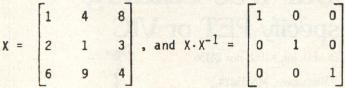To Calculate X-1: Tack onto matrix X an identity matrix of equal size: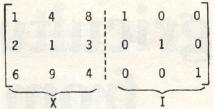The dotted line separates or partitions what is now a 3 row × 6 column matrix into two smaller matrices of equal size, X and I. The partition, in this case, is like the equator: it enables us to talk more easily about the halves of a whole. Is the matrix nearly inverted? "Not yet, not yet!" the Rabbit hastily interrupted. "There's a great deal to come before that!"1 Namely: Transform the left portion of the 3 × 6 matrix into I, using row and column operations on the entire matrix to do this. What is initially the identity matrix will emerge as X-1. In more detail, the following three steps are performed for as many times (iterations) as there are rows in X: Search would-be key elements, illustrated below, for the one of highest absolute value. Place this element in the pivot position, if it is not already there, by switching rows or columns. The pivot position for iteration #1 is the first spot in the principal diagonal, going from upper left to lower right. For iteration #2 it is the second spot, and so on. Keep track of columns that switch place, and interchange corresponding rows in the next-to-final form inverted matrix. Make the diagonal element in a column equal to 1, and make the other elements in the same column equal to 0. For example: Step 1:Would-be key elements are circled.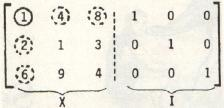Module 2 in the computer program tacks I onto X. The solid circle is the position to pivot on in the first iteration. Would-be key elements, besides 1, are in the dashed circles. They are the members of X that can be moved into the pivot position by switching a row or column. Module 3 identifies 8 as the would-be key element of highest absolute value. Step 2: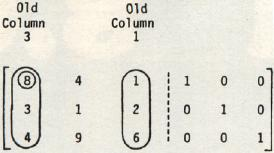To place 8 in the pivot position, columns 1 an 3 are switched (lines 3120 and 3130). Vector M "remembers" the column interchange.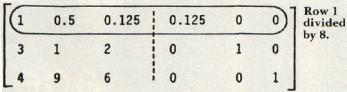Step 3: The pivot element becomes 1 by dividing the first row in the entire matrix by 8 (lines 3140 to 3190). Next, the second and third elements in column 1 become 0's: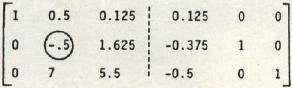This is done by multiplying row 1 by -3 and adding the product to row 2, and by multiplying row 1 by -4 and adding the product to row 3. Lines 3200 to 3270 accomplish this. This completes the first cycle of steps 1, 2, and 3. The circle is the new position to pivot on. The process continues as before. Skipping a few steps: Step: Pentultimate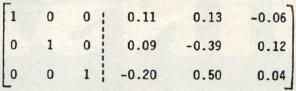This matrix finally emerges. Recall, however, that columns 1 and 3 were interchanged in the second step of the first cycle. Hence, rows 1 and 3 must now be switched (module 4): Step: Last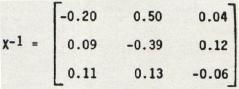Module 5 is easily changed to display X-1 differently, e.g., to show more decimal places or to accommodate a large number of rows and columns, say 8 or more. Finally, as an example of the routine's speed, inverting a 10 by 10 identity matrix takes about 45 to 50 seconds. This is not very fast. However, some of the algorithm's time is spent checking columns for the highest key element and for switching rows, when necessary, in the next-to-final-form inverted matrix. Some programs omit this check. But when they do, a danger arises that X will not be inverted when it really can be. And further, the elements of the inverted matrix may be of lessened precision. The preference here is for effectiveness rather than speed. But Gauss, always the perfectionist whose motto was "Few, but ripe," might challenge us: "Can't we have both?" 1Lewis Carroll, Alice's Adventures in Wonderland.